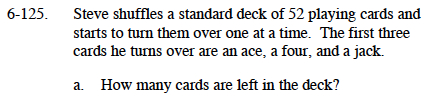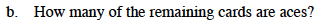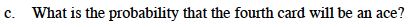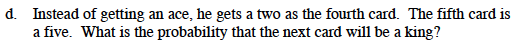### Home > MC1 > Chapter 6 > Lesson 6.3.1 > Problem6-125

6-125.Subtract the number of cards he has turned over from the total number in the deck.

49How many aces are there in a deck of cards? How many aces has Steve already turned over?You will need to use your answers from parts (a) and (b) to solve this problem.

$\frac{\text{number of aces left}}{\text{number of cards left}} = \frac{3}{49}$After Steve removes 5 cards, how many cards are left in the deck? How many of those cards are kings?